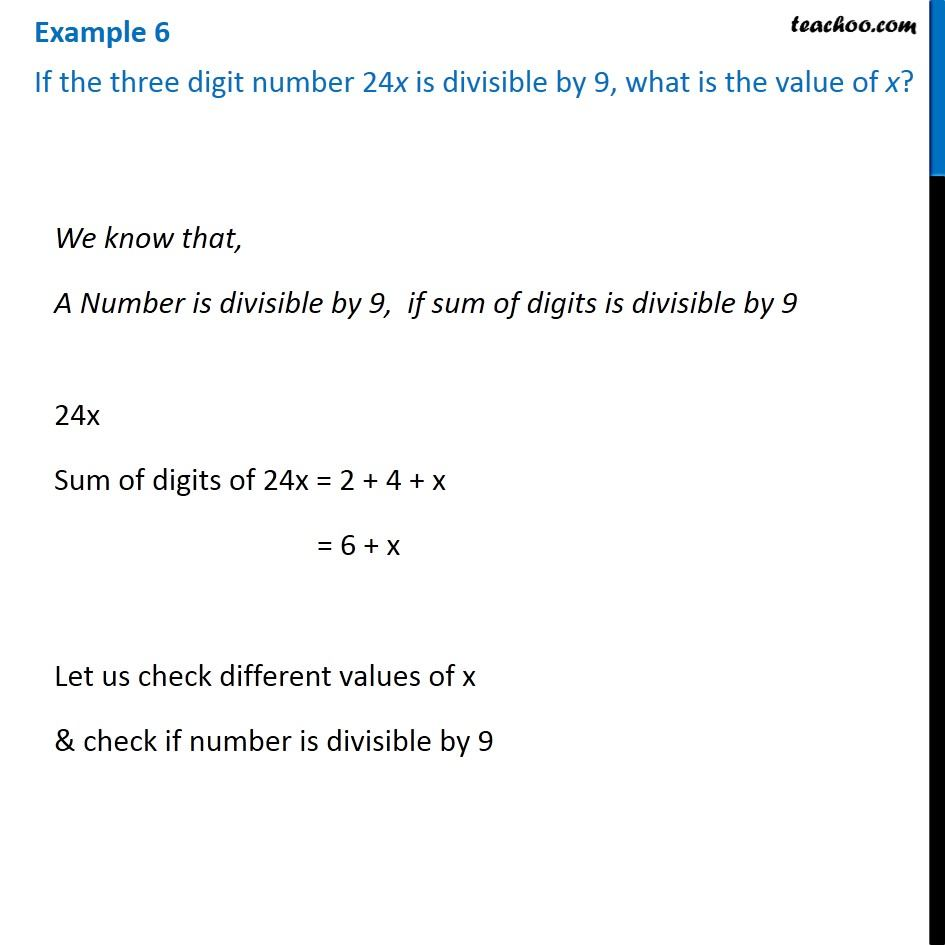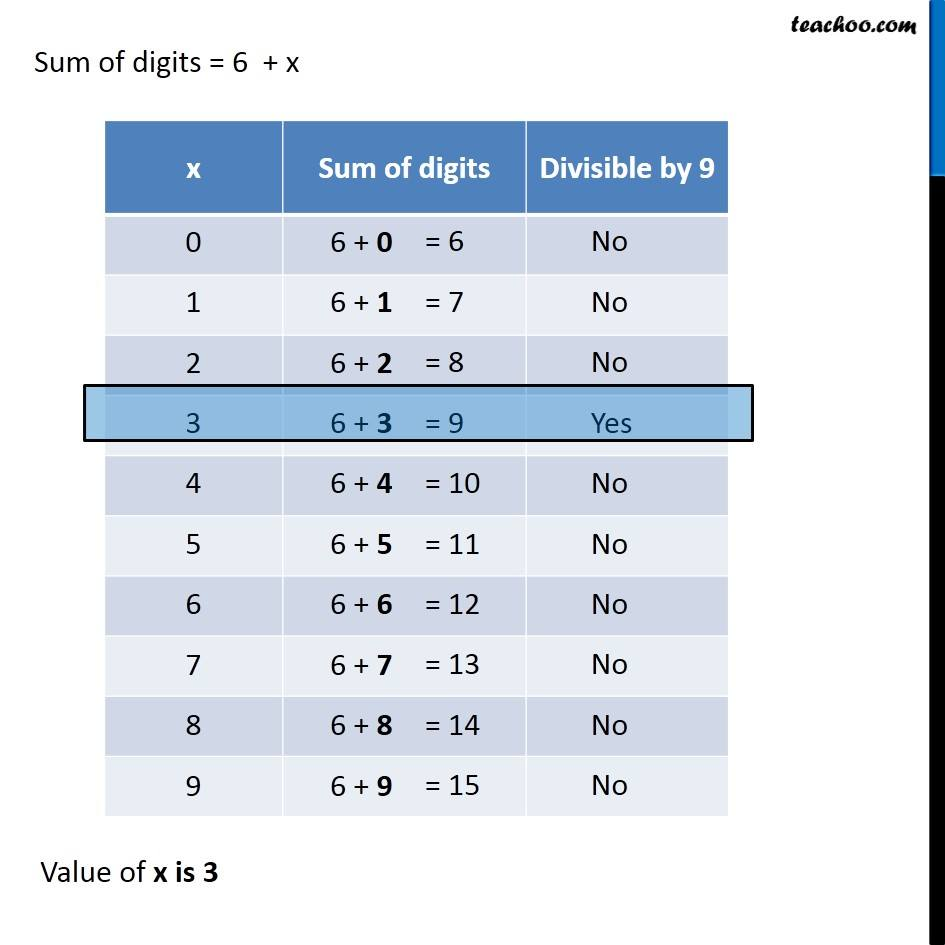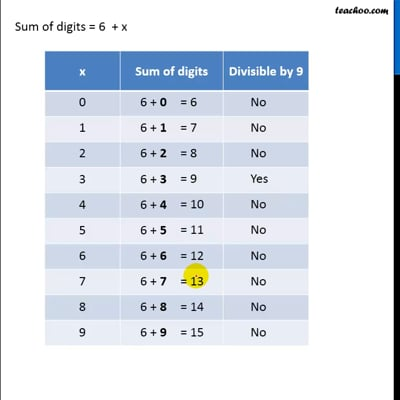Examples

Chapter 16 Class 8 Playing with Numbers
Serial order wiseThis video is only available for Teachoo black users

### Transcript

Sum of digits = 1 + 5 + 2 + 8 + 7 + 5 = 28 Since 28 is not divisible by 9, ∴ 152875 is also not divisible by 9. We know that, A Number is divisible by 9, if sum of digits is divisible by 9 24x Sum of digits of 24x = 2 + 4 + x = 6 + x Let us check different values of x & check if number is divisible by 9 Sum of digits = 6 + x Sum of digits = 6 + x Value of x is 3